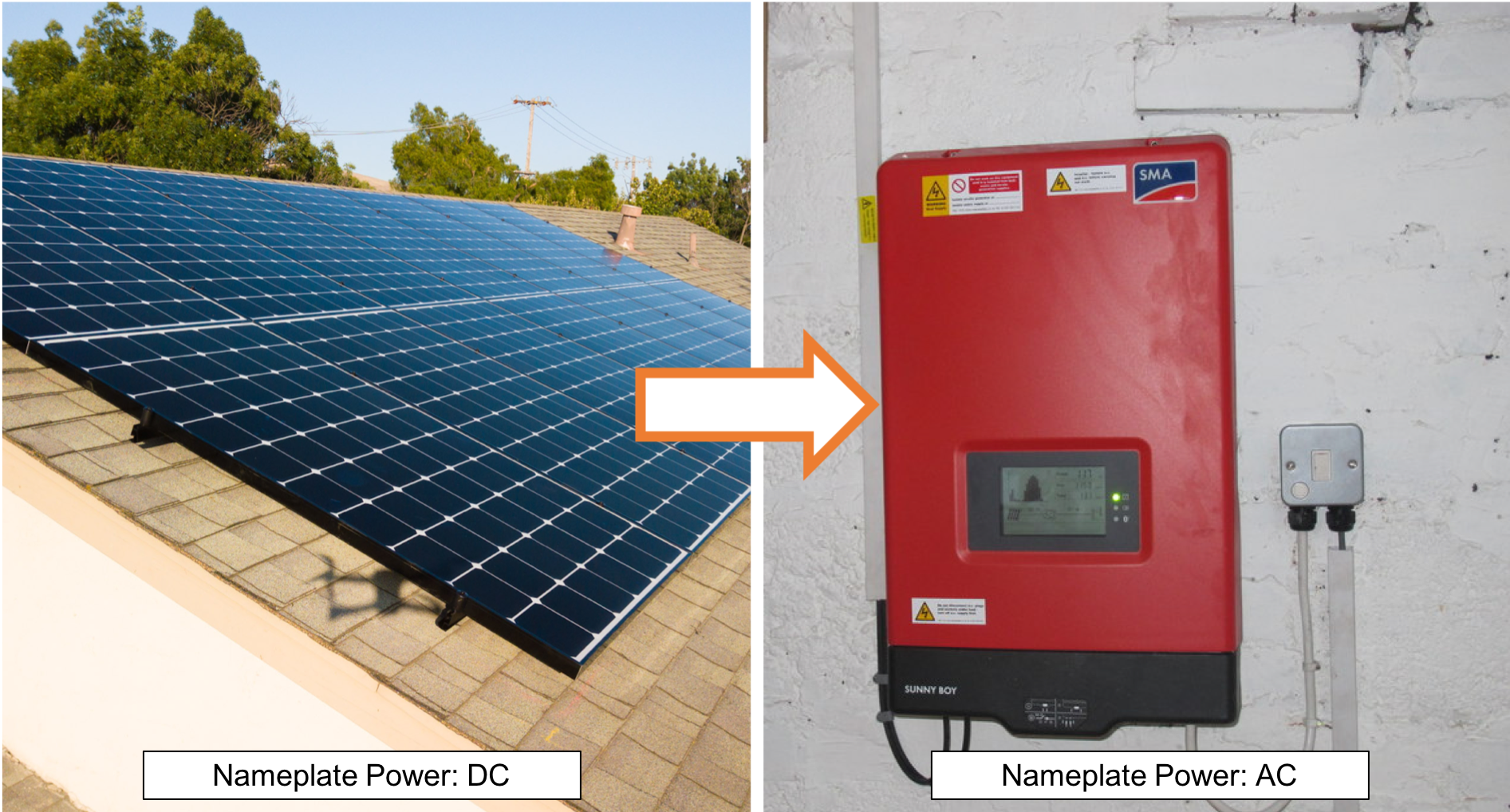# Understanding DC/AC Ratio

A common source of confusion in designing solar systems is the relationship between the PV modules, inverter(s), and their "nameplate" power ratings.

You will often see a system designed with a PV system with a power rating greater than the power rating of the inverter. For example, it would be common to see a 9 kW direct current (DC) module system paired with a 7.6 kW alternative current (AC) inverter. At first glance it may seem like the inverter is undersized and thus a limiting factor in the system creating power, but it actually a healthy ratio of PV power to inverter power.

Let’s look into detail as to why this is the case.### Nameplate DC Power is not the same as Nameplate AC Power

The only power generating component of the system is the PV array (the modules, also known as the DC power). For example a 9 kW DC PV array is rated to have the capacity to produce 9 kW of power at standard testing conditions (STC). STC is 1,000 W/m^2 and 25°C, and is more ideal than typical real world conditions. Thus the solar system will only produce at the full capacity of 9 kW on rare occasions, if ever, with most days being much less.

### Modules produce, inverters process

The inverter has the sole purpose of converting the electricity produced by the PV array from DC to AC so that the electricity can be usable at the property. Thus the nameplate rating of the inverter is its capacity to process the power of the PV array. For example a 7.6 kW inverter can produce an output of up to 7.6 kW AC.

### A 9KW array is rarely a 9KW power producer

A 9 kW DC solar array rarely produces this much power. The chart below actually shows ~4500 operating hours for a standard solar array, with each hour represented as a thin vertical slice. Note how rarely the array produces above 80% or 90% of the modules' rated DC power.Because the PV array rarely produces power to its STC capacity, it is common practice and often economically advantageous to size the inverter to be less than the PV array. This ratio of PV to inverter power is measured as the DC/AC ratio. A healthy design will typically have a DC/AC ratio of 1.25. The reason for this is that about less than 1% of the energy produced by the PV array throughout its life will be at a power above 80% capacity. Thus a 9 kW PV array paired with a 7.6 kW AC inverter would have an ideal DC/AC ratio with minimal power loss.

### Clipping Losses and DC/AC Ratio

When the DC/AC ratio of a solar system is too high, the likelihood of the PV array producing more power than the inverter can handle is increases. In the event that the PV array outputs more energy than the inverter can handle, the inverter will reduce the voltage of the electricity and drop the power output. This loss in power is known as “clipping”. For example, a DC/AC ratio of 1.5 will likely see clipping losses of 2-5%. Not as major as other losses, but still a noticeable effect.### What happens when I add more AC capacity (DC/AC < 1)?

Unless there are clipping losses, increasing the inverter size without increasing the modules capacity will not result in more energy output. In many cases, a 9 kW DC array of modules with a 7.6 kW AC inverter will produce an equal amount of power to pairing the array with a 10 kW AC inverter. With an oversized inverter you will have more capacity to convert DC to AC, but unless you plan to add more PV at a later date, the oversized inverter would likely be an unnecessary purchase.

 Scenario Standard Aggressive Conservative PV Array Size 9 kW 9 kW 9 kW Inverter Size 7.6 kW 6 kW 10 kW DC/AC Ratio 1.18 1.50 0.90 Clipping Loss 0.1% 2.2% 0.1% Energy Output 13,882 kWh 13,582 kWh 13,882 kWh

In conclusion, it is common practice to have as high of a DC/AC ratio as possible without having significant clipping loss.